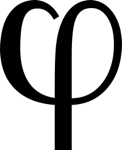1

## Fun With Phi

Published on Thursday, August 12, 2010 in , , , , , , ,I've talked quite a bit about Pi on this blog, but today, I'm turning my attention to a different number: phi. Like Pi, phi goes on forever, and can only be approximated. It's equal to roughly 1.6180339887, but is also known as the golden ratio.

What makes phi as a number so special? And what's with the name golden ratio, anyway? Of course, Grey Matters readers have an additional question: What kind of fun can we have with it?

### The Basics

Let's start with a quick video history of phi, its discovery, and its original uses:

Let's try making a column of 10 numbers in a Fibonacci sequence, using the same process mentioned in the above video. Have someone choose any two numbers, both below 10, to help keep the numbers manageable. As an example, let's choose 8 and 3. Let's see...8 and 3 make 11, 3 and 11 make 14, 11 and 14 make 25...if we continue, we wind up with this column of numbers:
```  8
3
11
14
25
39
64
103
167
270
```
How fast do you think you could find the sum of these numbers? It wouldn't take too long with a calculator, but what if you could add these numbers in your head quicker than a calculator?

How? Find the 7th number from the top (4th from the bottom), and simply multiply it by 11! In this case, the 7th number is 64, so we multiply 64 times 11 and get 704!

At this point, you probably have at least one of two questions. First, how do I multiply by 11 mentally? It's easier than you might think. Here's a video showing you how to multiply by 11 with up to 5 digit numbers:

For a further explanation, as well as some practice exercises, check out Math World's Math Tricks: Multiply by 11 page.

The other question you probably have is, “Why does multiplying the 7th number by 11 always work?” The surprisingly simple explanation is given here by Dr. Math.

One common addition to this trick is to have people divide the 10th number in the column by the 9th number, which you can also do without a calculator. In the example above, it would be 270/167, which is 1.6167664671. Look familiar? It's an approximation of phi! Thanks to the pattern imposed by the Fibonacci, you can always look like you're mentally calculating, say “1.61”, and you have one routine that makes you look like a mathematical genius!

Here's a game known as Corner The Lady (Java required), invented around 1960 by Rufus P. Isaacs. The rules are described at the link, so read them carefully. I suggest starting with the smallest board, which is 4 by 4, and working your way up as you discover how to win.

Hmmm...the title on the page reads Wythoff's Nim. Perhaps this is a form of Nim, and therefore always winnable with the proper strategy? Yep!

Technically, Corner The Lady and Wythoff's Nim are two different games. Wythoff's Nim is played with two piles of objects, each with a different number of objects. Like standard Nim, you can take as many matches as you want from either pile on your turn, and the object is to take the last remaining object. However, in Wythoff's Nim you can also take objects from both piles on your turn, with the restriction that you have to take the same number of objects from both piles.

In chapter 8 of Martin Gardner's book, Penrose Tiles to Trapdoor Ciphers, he points out the surprising fact these two games have exactly the same winning strategy, because they're mathematically the exact same game! Much of that chapter is reprinted here online, in case the chapter link at the start of this paragraph ceases to work.

So why is this included in a post on phi? It turns out that the safe positions (that is, the positions which will guarantee you a later win, assuming the goal square to be 0,0) can be generated using phi! If you want to find the N safe position, you multiply N by phi, round it down to the nearest whole number, and you've got your first coordinate. How do you get the second coordinate of a safe position? Simply add N to the first coordinate!

For example, if we're looking for the 1st pair of coordinates that's safe, we work through this way. N=1 (for the 1st pair), and N times 1.61 equals 1.61, which is rounded down to 1. That 1 is our first coordinate. 1 plus 1 = 2, so 2 is our second coordinate.

So, now we have 1 and 2, does that mean our first safe square is plotted at 1,2 or 2,1? Surprisingly, both are correct! The formula we used actually generates a pair of safe squares each time you use it. Isn't phi nice? It gives us a formula with which it's hard to make a mistake.

Where do we go from here? I suggest taking a look around the web, including images and videos, and searching mainly for the terms Fibonacci and golden ratio. There's so much more to discover about these fascinating concepts. Phi is one of those ratios, like Pi, that pops up in the most surprising of places.

### 1 Response to Fun With Phijw

Never knew about dividing the last 2 numbers in the sequence... one more thing to add. Excellent!